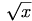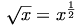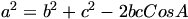Warning: Illegal string offset 'html' in /home/hsn/public_html/forum/cache/skin_cache/cacheid_1/skin_topic.php on line 909

Maths Help - HSN forum# Maths Help

9 replies to this topic

### #1Rocky

• Gender:Male

Posted 17 November 2005 - 05:01 PM

1- Differentiate:
y=(2x+3)(squared) OVER 2x

*Not working out for me, so help would be appreciated

2- Find the Max. and Min. value of f(x) in the interval [1,3] where
f(x)=x(cubed) - 6x

*How do you do that Q?

### #2Richard_05

Child Prodigy

•• Members
•• 637 posts
• Interests:I'm not going to sit and type my grades, nor my plans for the future, nor a random fact. :)
• Gender:Male

Posted 17 November 2005 - 05:39 PM

Well, for the first one it would be handy to remember the a^2 + 2ab + b^2 rule, and remember to change square root to index form, maybe that helps?

### #3Rocky

• Gender:Male

Posted 17 November 2005 - 06:28 PM

I opened the brackets, though some of the fractions don't make sense, so what answer did you get when you did it?

### #4dfx

Fully Fledged Genius

•• Members
•• 1,955 posts
• Gender:Male

Posted 17 November 2005 - 06:33 PM

Expand the brackets on the numerator. Split the fraction so that you have each term with thein the denominator. The usingu can then the subtract the powers of the numerator and denominator as you're dividing and get it into linear form. Then it's just straightforward.

Post up your final answer if you need it checked... try to show working, though I know it's a little tough if you don't know how to use the latex coding.

### #5Rocky

• Gender:Male

Posted 17 November 2005 - 06:40 PM

Ok, I'll post later what I got. As busy working on my English H.W.

### #6Rocky

• Gender:Male

Posted 18 November 2005 - 03:35 PM

I have solved both Qs, myself but can someone atleast help me with this Q:

Solve the Eqn 2cos2x+cosx-1=0, where 0<=x<=360 (degrees)

*As I am not good with Addition Formulae, so can someone help me get it in a form for me to solve.

### #7The Wedge Effect

HSN Legend

•• Members
•• 2,477 posts
• Location:Paisley
• Gender:Male

Posted 18 November 2005 - 04:16 PM

QUOTE(Rocky @ Nov 18 2005, 03:35 PM)
I have solved both Qs, myself but can someone atleast help me with this Q:

Solve the Eqn 2cos2x+cosx-1=0, where 0<=x<=360 (degrees)

*As I am not good with Addition Formulae, so can someone help me get it in a form for me to solve.Yeah, cos2x would be expanded using the double angle formulae into 2 cos²x - 1, so for 2cos2x, you'd get 2(2 cos²x - 1).

When you simplify, you get a quadratic of the form ax² + bx +c=0:

4cos² x + cos x -3=0

Let cos x = y

4y² + y - 3 =0

(4y-3)(y+1)=0you get:

(4cos x - 3)(cos x + 1) = 0

I'm sure you can work out the answers from that.### #8Rocky

• Gender:Male

Posted 18 November 2005 - 04:31 PM

Thanks dude.

### #9Rocky

• Gender:Male

Posted 19 November 2005 - 02:13 PM

Anyone know how to do this:

Theres a triangle, "<|" And the bottom side is 4cm. And the 2 sloped sides are 3cm. The top point has an angle X. I halved it so it'd be X/2 angle and got Sin=2/3, but not an exact value so anyone got any idea?

*As the Q is:
Find the exact value of sinX.

### #10dfx

Fully Fledged Genius

•• Members
•• 1,955 posts
• Gender:Male

Posted 19 November 2005 - 02:19 PM

Cosine rule?Where a, b and c are the three sides, and A is the angle opposite side A. So rearrange for A and plug in a,b,c and get your value. (In this case obviously you would have x and X as a and A.)

#### 1 user(s) are reading this topic

0 members, 1 guests, 0 anonymous users